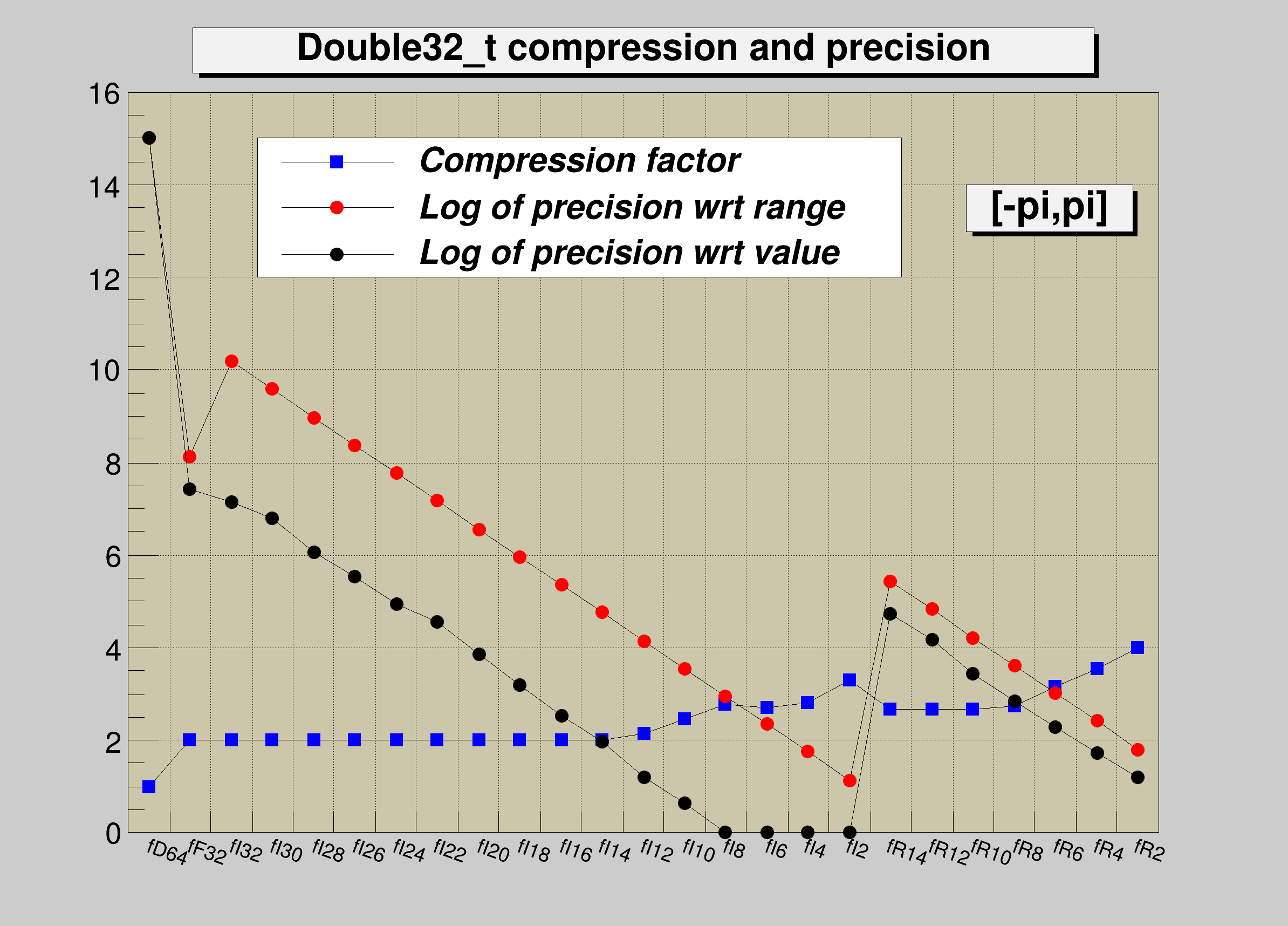ROOT   6.14/05 Reference Guide
double32.C File Reference

## Detailed DescriptionTutorial illustrating use and precision of the Double32_t data type You must run this tutorial with ACLIC: a dictionary will be automatically created.

root > .x double32.C+

The following cases are supported for streaming a Double32_t type depending on the range declaration in the comment field of the data member:

Case Declaration
A Double32_t fNormal;
B Double32_t fTemperature; //[0,100]
C Double32_t fCharge; //[-1,1,2]
D Double32_t fVertex; //[-30,30,10]
E Double32_t fChi2; //[0,0,6]
F Int_t fNsp;
Double32_t* fPointValue; //[fNsp][0,3]
• Case A fNormal is converted from a Double_t to a Float_t
• Case B fTemperature is converted to a 32 bit unsigned integer
• Case C fCharge is converted to a 2 bits unsigned integer
• Case D the array elements of fVertex are converted to an unsigned 10 bits integer
• Case E fChi2 is converted to a Float_t with truncated precision at 6 bits
• Case F the fNsp elements of array fPointvalue are converted to an unsigned 32 bit integer. Note that the range specifier must follow the dimension specifier.

Case B has more precision than case A: 9 to 10 significative digits and 6 to 7 digits respectively. The range specifier has the general format: [xmin,xmax] or [xmin,xmax,nbits]. Examples

• [0,1]
• [-10,100];
• [-pi,pi], [-pi/2,pi/4],[-2pi,2*pi]
• [-10,100,16]
• [0,0,8] Note that:
• If nbits is not specified, or nbits <2 or nbits>32 it is set to 32
• If (xmin==0 and xmax==0 and nbits <=14) the double word will be converted to a float and its mantissa truncated to nbits significative bits.

## IMPORTANT NOTE

Lets assume an original variable double x. When using the format 0,0,8 you get the best relative precision when storing and reading back the truncated x, say xt. The variance of (x-xt)/x will be better than when specifying a range for the same number of bits. However the precision relative to the range (x-xt)/(xmax-xmin) will be worst, and vice-versa. The format [0,0,8] is also interesting when the range of x is infinite or unknown.#include "TFile.h"
#include "TCanvas.h"
#include "TTree.h"
#include "TH1.h"
#include "TMath.h"
#include "TRandom3.h"
#include "TGraph.h"
#include "TLegend.h"
#include "TFrame.h"
#include "TPaveLabel.h"
class DemoDouble32 {
private:
Double_t fD64; //reference member with full double precision
Double32_t fF32; //saved as a 32 bit Float_t
Double32_t fI32; //[-pi,pi] saved as a 32 bit unsigned int
Double32_t fI30; //[-pi,pi,30] saved as a 30 bit unsigned int
Double32_t fI28; //[-pi,pi,28] saved as a 28 bit unsigned int
Double32_t fI26; //[-pi,pi,26] saved as a 26 bit unsigned int
Double32_t fI24; //[-pi,pi,24] saved as a 24 bit unsigned int
Double32_t fI22; //[-pi,pi,22] saved as a 22 bit unsigned int
Double32_t fI20; //[-pi,pi,20] saved as a 20 bit unsigned int
Double32_t fI18; //[-pi,pi,18] saved as a 18 bit unsigned int
Double32_t fI16; //[-pi,pi,16] saved as a 16 bit unsigned int
Double32_t fI14; //[-pi,pi,14] saved as a 14 bit unsigned int
Double32_t fI12; //[-pi,pi,12] saved as a 12 bit unsigned int
Double32_t fI10; //[-pi,pi,10] saved as a 10 bit unsigned int
Double32_t fI8; //[-pi,pi, 8] saved as a 8 bit unsigned int
Double32_t fI6; //[-pi,pi, 6] saved as a 6 bit unsigned int
Double32_t fI4; //[-pi,pi, 4] saved as a 4 bit unsigned int
Double32_t fI2; //[-pi,pi, 2] saved as a 2 bit unsigned int
Double32_t fR14; //[0, 0, 14] saved as a 32 bit float with a 14 bits mantissa
Double32_t fR12; //[0, 0, 12] saved as a 32 bit float with a 12 bits mantissa
Double32_t fR10; //[0, 0, 10] saved as a 32 bit float with a 10 bits mantissa
Double32_t fR8; //[0, 0, 8] saved as a 32 bit float with a 8 bits mantissa
Double32_t fR6; //[0, 0, 6] saved as a 32 bit float with a 6 bits mantissa
Double32_t fR4; //[0, 0, 4] saved as a 32 bit float with a 4 bits mantissa
Double32_t fR2; //[0, 0, 2] saved as a 32 bit float with a 2 bits mantissa
public:
DemoDouble32() {;}
void Set(Double_t ref);
};
void DemoDouble32::Set(Double_t ref) {
fD64 = fF32 = fI32 = fI30 = fI28 = fI26 = fI24 = fI22 = fI20 = ref;
fI18 = fI16 = fI14 = fI12 = fI10 = fI8 = fI6 = fI4 = fI2 = ref;
fR14 = fR12 = fR10 = fR8 = fR6 = fR4 = fR2 = ref;
}
void double32() {
// show the use and precision of the Double32_t data type
DemoDouble32 *d = new DemoDouble32();
//create a Tree with 40000 objects DemoDouble32
TFile::Open("DemoDouble32.root","recreate");
TTree *T = new TTree("T","DemoDouble32");
TBranch *bd = T->Branch("d","DemoDouble32",&d,4000);
Double_t xmax = TMath::Pi();
Double_t xmin = -xmax;
Int_t i, n = 40000;
for (i=0;i<n;i++) {
d->Set(r.Uniform(xmin,xmax));
T->Fill();
}
T->Write();
//Create the frame histogram and the graphs
Int_t nb = branches->GetEntries();
TBranch *br = (TBranch*)branches->At(0);
Long64_t zip64 = br->GetZipBytes();
Double_t cx = 1;
Double_t drange = 15;
Double_t dval = 15;
TCanvas *c1 = new TCanvas("c1","c1",800,600);
c1->SetGrid();
c1->SetFillColor(17);
TH1F *h = new TH1F("h","",nb,0,nb);
h->SetMaximum(16);
h->SetStats(0);
h->Draw();
c1->GetFrame()->SetFillColor(21);
c1->GetFrame()->SetBorderSize(12);
TGraph *gcx = new TGraph(nb); gcx->SetName("gcx");
gcx->SetMarkerStyle(21);
gcx->SetMarkerColor(kBlue);
TGraph *gdrange = new TGraph(nb); gdrange->SetName("gdrange");
gdrange->SetMarkerStyle(20);
gdrange->SetMarkerColor(kRed);
TGraph *gdval = new TGraph(nb); gdval->SetName("gdval");
gdval->SetMarkerStyle(20);
TPaveLabel *title = new TPaveLabel(.15,.92,.85,.97,"Double32_t compression and precision","brNDC");
title->Draw();
//loop on branches to get the precision and compression factors
for (i=0;i<nb;i++) {
br = (TBranch*)branches->At(i);
h->GetXaxis()->SetBinLabel(i+1,br->GetName());
cx = Double_t(zip64)/Double_t(br->GetZipBytes());
gcx->SetPoint(i,i+0.5,cx);
if (i > 0) {
T->Draw(Form("(fD64-%s)/(%g)",br->GetName(),xmax-xmin),"","goff");
Double_t rms = TMath::RMS(n,T->GetV1());
drange = TMath::Max(0.,-TMath::Log10(rms));
}
gdrange->SetPoint(i,i+0.5,drange);
if (i > 0) {
T->Draw(Form("(fD64-%s)/(fD64+0.01)",br->GetName()),"","goff");
Double_t rms = TMath::RMS(n,T->GetV1());
dval = TMath::Max(0.,-TMath::Log10(rms));
}
gdval->SetPoint(i,i+0.5,dval);
}
gcx->Draw("lp");
gdrange->Draw("lp");
gdval->Draw("lp");
TLegend *legend = new TLegend(0.2,0.7,0.7,0.85);
legend->SetTextFont(72);
legend->SetTextSize(0.04);